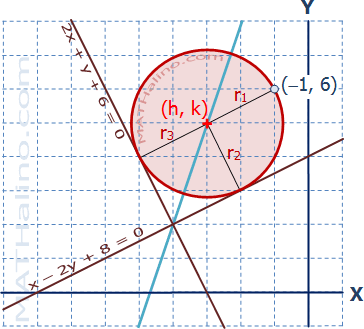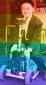# find the equation of a circle passing through (-1,6) and tangent to lines $x-2y+8=0$ and $2x+y+6=0$

3 posts / 0 new
Elbert Delos Santosfind the equation of a circle passing through (-1,6) and tangent to lines $x-2y+8=0$ and $2x+y+6=0$
Tags:
Jhun VertLet

• r1 = distance between (h, k) and (-1, 6)
• r2 = distance between (h, k) and x - 2y + 8 = 0
• r3 = distance between (h, k) and 2x + y + 6 = 0
• r = r1 = r2 = r3$r_1 = \sqrt{(h + 1)^2 + (k - 6)^2}$

$r_2 = \dfrac{h - 2k + 8}{(-)(+)\sqrt{1^2 + (-2)^2}} = \dfrac{h - 2k + 8}{-\sqrt{5}}$

$r_3 = \dfrac{2h + k + 6}{(+)(+)\sqrt{2^2 + 1^2}} = \dfrac{2h + k + 6}{\sqrt{5}}$

$r_2 = r_3$

$\dfrac{h - 2k + 8}{-\sqrt{5}} = \dfrac{2h + k + 6}{\sqrt{5}}$

$-h + 2k - 8 = 2h + k + 6$

$k = 3h + 14$

$r_1 = r_3$

${r_1}^2 = {r_3}^2$

$(h + 1)^2 + (k - 6)^2 = \dfrac{(2h + k + 6)^2}{5}$

Substitute k = 3h + 14
$(h + 1)^2 + (3h + 14 - 6)^2 = \dfrac{(2h + 3h + 14 + 6)^2}{5}$

$(h + 1)^2 + (3h + 8)^2 = \dfrac{(5h + 20)^2}{5}$

$(h^2 + 2h + 1) + (9h^2 + 48h + 64)^2 = \dfrac{25h^2 + 200h + 400}{5}$

$10h^2 + 50h + 65 = 5h^2 + 40h + 80$

$5h^2 + 10h - 15 = 0$

$h^2 + 2h - 3 = 0$

$(h - 1)(h + 3) = 0$

$h = 1 ~ \text{and} ~ -3$

When h = 1

$k = 3(1) + 14 = 17$

$r^2 = (1 + 1)^2 + (17 - 6)^2$

$(x - 1)^2 + (y - 17)^2 = 125$           answer

When h = -3

$k = 3(-3) + 14 = 5$

$r^2 = (-3 + 1)^2 + (5 - 6)^2$

$(x + 3)^2 + (y - 5)^2 = 5$           answer

Michael LeungRealize the two lines are perpendicular.
Intersection of the lines, by isolating and equating y:
x/2+4=-2x-6
(-4,2)
By trig, find slope of bisector of the two lines is 3.
Equation of bisector in point-slope form is 3=(y-2)/(x+4) => k=3h+14 {1}
Distance from center of circle to (-4,2) is sqrt(2)*Distance from center of circle to (-1,6)
(h+4)2+(k-2)2=2*((h+1)2+(k-6)2)
Simplify and sub in {1}
0=h2+2h-3
h=-3 or 1, k=5 or 17, r2=25 or 125 by back substitution

• Mathematics inside the configured delimiters is rendered by MathJax. The default math delimiters are $$...$$ and $...$ for displayed mathematics, and $...$ and $...$ for in-line mathematics.